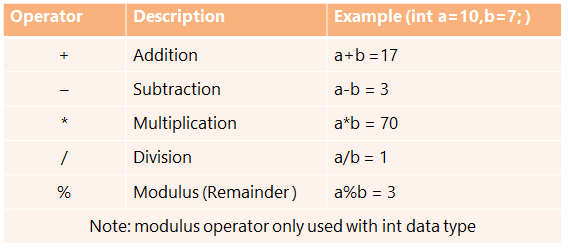Arithmetic Operators

Arithmetic operators are used to evaluate mathematical expressions. Arithmetic operators are a form of operator that may either by a Unary Operator or a Binary Operator.Example Programs

Program 

Write a program that calculates the number of miles that light will travel in 1000 days, which illustrates the use of long integer.

Program 

Create a program that will generate b bill at McDonald’s for four vegetable burgers (@Rs 45 per vegetable Burger) and three vegetable McPuffs(@Rs 25 per vegetable McPuff). There is a special Independence Day discount of Rs 50 on the final bill amount.

Program 

Write a program to find the sum and difference between 25 and 16 using variables in different lines.

Program 

Write a program to input the length and breadth of a rectangle and find its area and perimeter.

Note:

Program 

Write a java program to accept the radius and height of cone and calculate the volume of a cone.

Program 

Write a program to initialize three int variables a, b and c with 234, 456 and 712 and store the sum of the last digits of the variables into d and display it.

Program 

Write a program to input five integers and find their average.

Program 

Write a program to input the Principal, Rate and Time for a certain amount of money and print the Simple Interest.

Program 

Write a program to input the length and breadth of a rectangle and find its area and perimeter.

Program 

Write a program to input the radius of a circle and find its area and perimeter.

Note: Area of a circle=22/7* radius2

Circumference of a circle= 2 * 22/7 * radius

Program 

Write a program to input the length, breadth and height of a cuboid and find its Volume and Total Surface Area.

Note: Volume of a cuboid= length*breadth*height

Program 

Write a program to input the radius and height of a cylinder and find its volume and total surface area.

Note: Total Surface Area=22 + 2h

Volume=2h

Program 

Write a program to input the temperature in Fahrenheit and change it to Celsius.

Note: The relation between Fahrenheit and Celsius is given by the formula:

Where C=Celsius and F=Fahrenheit

Program 

Write a program to input time in seconds and display the time broken up as hours, minutes and seconds.

For Example:

INPUT: Enter Time in Seconds: 4326

OUTPUT:

Time in hours:1

Time in minutes:12

Time in seconds:6

Program 

Write a program to input the time in hours, minutes and seconds and print it in seconds.

For Example:

INPUT: Enter time in hours:

1

Enter time in minutes:

12

Enter time in seconds:

6

OUTPUT:

Time in seconds:4326

Program 

Write a program to input three integers and find their sum, without using the mathematical operator +.

Program 

Write a program to input the Basic Pay of an employee and find the gross pay of the employee for the following allowances and deductions.

Dearness Allowance = 25% of Basic Pay

House Rent Allowance=15% of Basic Pay

Provident Fund=8.33% of Basic Pay

Net Pay=Basic Pay + Dearness Allowance + House Rent Allowance

Gross Pay= Net Pay – Provident Fund

Program 

Write a program to input an integer and if positive change it to negative and if negative change it to positive.

INPUT: Enter an integer: 12

OUTPUT: Sign changed:-12

INPUT: Enter an integer: -14

OUTPUT: Sign changed: 14

Program 

If the marks obtained by a student in five different subjects are input through the keyboard, write a program to find out the aggregate marks and percentage marks obtained by the student. Assume that the maximum marks that can be obtained by a student in each subject is 100.

Program 

If the total selling price of 15 items and the total profit earned on them is input through the

keyboard, write a program to find the cost price of one item.